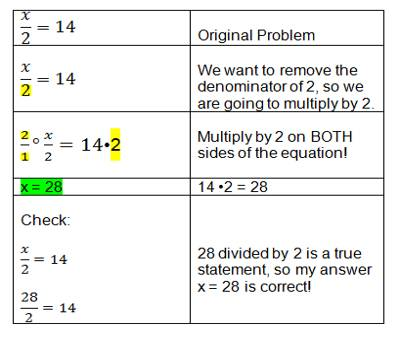Multiplication Equations

An equation is a mathematical statement such that the expression on the left side of the equals sign (=) has the same value as the expression on the right side. An example of an equation is 9 * 8 = 72.

One of the terms in an equation may not be known and needs to be determined. The unknown term may be represented by a letter such as x. (e.g. x * 8 = 72).

The solution of an equation is finding the value of the unknown x. Use the division property of equations to find the value of x. The division property of equations states that the two sides of an equation remain equal if both sides are divided by the same number

Example:

x * 5 = 10

x * 5 ÷ 5 = 10 ÷ 5

x * 1 = 2

x = 2

Check the answer by substituting the answer (2) back into the equation.

2 * 5 = 10.Explanation:

Mt. Everest in Nepal is the world’s tallest mountain, about 29,000 ft. high. It is twice as high as Mount Whitney in California. How high is Mount Whitney?

We can write a multiplication equation to find the answer to problems like this. Our unknown number is the height of Mount Whitney. Let x represent this height. We know that 2x is the height of Mount Everest. We can write our equation like this:

2x = 29,000 ft

To solve this equation, we can use the inverse of multiplying by 2, which is dividing by 2.

If we divide the left side of the equation by 2, we will get x alone on the left. Remember, any operation done to one side must also be done to the other side, so we must also divide the right side by 2.

We divide and find that x is equal to 14,500 ft. This is very close to the actual height of Mount Whitney, which is 14,494 ft.

Mental Math

You don’t always need to use the calculator or pencil and paper to solve equations. You can solve many of them mentally. Use your mental muscle on these problems! Come up with an answer on your own, before looking at the answers below

1. 10x = 350
2. (12)(5)n = 0
3. 6 + 6 = 3x
4. 19y = 1900